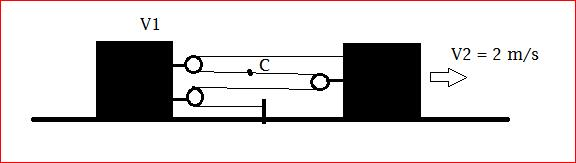# Find Velocity of Point CFind the velocity of point C on string.

•rahulsidhu ·

On further reflection, my answer is 1 m/s , to the right.

Using the constant string length, v1= 3/4 v2 = 1.5m/s. This can be seen in this way: the lowest part of string is decreasing at a rate v1, while the other 3 parts are increasing at (v2-v1) each.
3(v2-v1) = v1

Now, looking at the topmost pulley attached to block 1, the string on top is moving to the right at 2m/s, the pulley itself is moving at 1.5m/s, thus the string is slipping at 0.5m/s w.r.t the pulley. Similarly, the lower string is moving to the left at 0.5 m/s w.r.t to the pulley (this was my first answer). However, the velocity w.r.t a stationary observer is 1m/s, to the right.

•funkygp ·

i guess the ans is 3/2...........

just asssume the 1st block moves by x dist to the left.......then extra 3x dist is left to cover,which has to be covered by the body v1 and it has to be divided into 4 ways....

thus, the velocity of each segment of v1,

3x4=x'(the dist covered by body v1 ,which is connected by 4 segmnets,which has to be covered equally by each segments)

point c is on one of the segment attached to the v1 ......thus the vel of it will be x',

3*24=1.5m/s

well i guess i m not good at xplaining!!!!!
nyways this was my thought

regards,
funkygp

•rishabh ·

is it 2 m/s.
by constraint we cn find v1 = 34v2

•Vivek @ Born this Way ·

No it isn't I think!

•rahulsidhu ·

Is it 1/2 m/s?

•Vivek @ Born this Way ·

May be.. You may wish to post the solution.

•